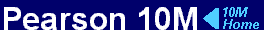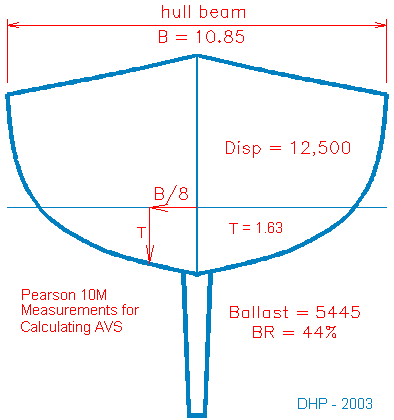AVS Calculation
AVS Calculation Back to ProjectsIn the 30th Anniversary edition of Adlard Coles Heavy Weather Sailing Peter Bruce offers a formula for the calculation of an approximation of the angle of vanishing stability (AVS) using readily available data on a boat. The formula is:

AVS = 110 + ( 400 / (SV-10) )
SV is the screening value and is calculated by:
SV = B2 / (R x T x V1/3)
Expanding SV in the first equation gives:
AVS = 110 + ( 400 / (B2 / (R x T x V1/3))-10) )

B = beam of the hull (no rub rail) in meters
R = ballast ratio
T = hull draft at B/8 from centerline in meters
V = displacement volume in cubed meters

 For the 10M these values are: B = 10.85 ft = 3.31 m R = .44 T = 1.63 ft = 0.50 m V = 12,500 lbs = 5.53 cubed metersAVS10M = 110 + ( 400 / (3.312 / (.44 x .5 x 5.531/3)) - 10 )
AVS10M = 131.6

By the formula the AVS for the 10M is 131.6 degrees. According to Bruce the formula is only an approximation and may be in error by as much as 15 degrees for some types. And indeed the AVS of 131.6 does not match with the measured LPS (limit of positive stability) for a particular 10M as shown on the USYRA stability calculation. The USYRA value of 123 degrees is lower by 8.5 degrees. The USYRA value is derived from tests and actual measurements of the boat. The AVS calculation is an estimate based on the actual test results on a number of "typical sailing yachts".

US Sailing has an AVS calculator on it's web site where the data may be entered to find the AVS using the formula from Heavy Weather Sailing. On the web site they use a measurement for T (hull draft) that does not match with the formula in the book. They take it as simply hull draft rather than the depth at B/8 outboard from centerline. This will result in a larger value for T and thus a larger value for AVS. The difference for the 10M with the US Sailing value for T and the books value is an AVS of 135.1 vs 131.6. That's a pretty big difference and could move a boat from one race qualification class to another.

It should also be kept in mind that the AVS is a static measure of stability for a boat at rest in flat (calm) water. The angle alone is an incomplete assessment. The stability curve ( a curve of righting moment vs angle of heel) will show the relative stability of an upright hull vs inverted. This can indicate a boats ability to recover from capsize. The dynamics of a boat in motion in a seaway have large effects on stability. Any proper evaluation of a boats suitability to offshore sailing in rough ocean conditions must account for more than just AVS.

Reference: Bruce, Peter (1999) Adlard Coles' Heavy Weather Sailing 30th Anniversary Ed, International Marine

USYRA stability data for the 10M
US Sailing AVS Calculator
Graham Radford Yacht Design, STABILITY DISCUSSION